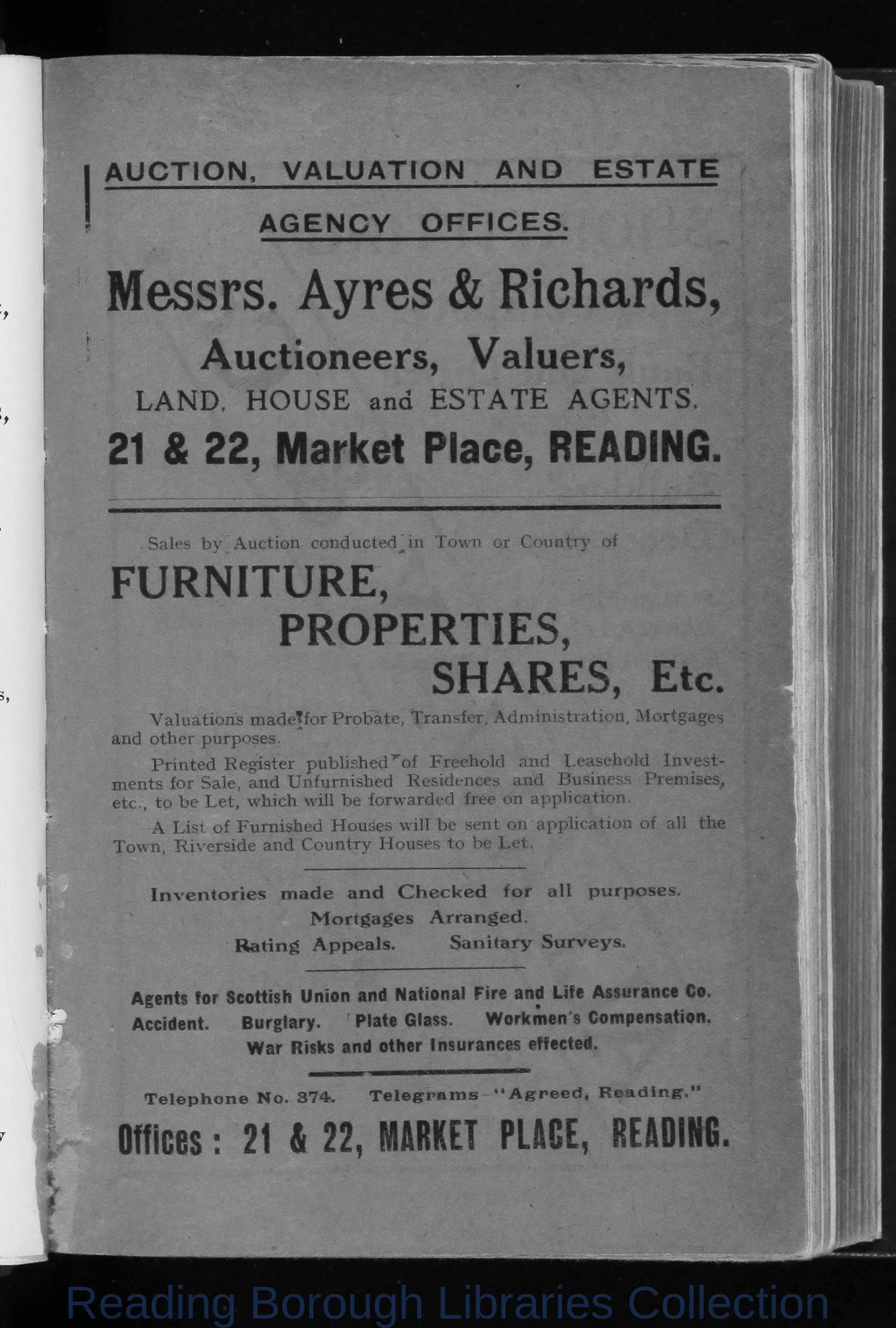## Image Details

There is no information available.

## OCR Text

A U C T IO N ,

V A L U A T IO N
AGENCY

AND

ESTATE

O F F IC E S .

M e s s r s . A y r e s & R ic h a r d s ,
A u ctio n eers,

V alu e rs,

LA N D . H O U SE and E S T A T E A G E N T S.

21 & 22, Market Place, READING.
S a le s

b y

A u c tio n

co n d u cte d

in

Tow n

or

C o u n try

of

F U R N IT U R E ,
P R O P E R T IE S,
SH ARES,

Etc.

V a lu a t io n s m a d e T fo r P r o b a te , T r a n s fe r , A d m in is tr a t io n , M o r tg a g e s
and

o th e r p u rp o se s.
P r in te d

R e g is te r

m e n ts fo r S a le , a n d

A
T ow n ,

p u b l i s h e d â' o f

U n fu r n is h e d

e tc ., t o b e L e t , w h ic h

L is t o f F u r n is h e d
R iv e r s id e

an d

F r e e h o ld

R e s id e n c e s

w ill b e fo r w a r d e d
H o u s e s w ill b e

C o u n tr y H o u se s to

and
an d

L e a s e h o ld
B u s in e s s

fr e e o n

a p p lic a tio n .

sen t on

a p p lic a tio n

In v e stÂ­

P r e m is e s ,

o f

a ll

th e

b e L e t,

In ven tories m ade and C h e c k e d

for

all

purposes.

M ortgages Arranged.
R ating Appeals.

Sanitary Surveys.

A g e n ts fo r S co ttish U n io n a nd N a tio n a l F ir e a n d L ife A s s u ra n c e C o .
A c c id e n t.

B u r g la r y .

P late G lass.

W o r k m e n s C o m p e n s a tio n .

W a r R isks a n d o th e r In s u ra n c e s effected.

T e le p h o n e N o. 374.

T e le g r a m s

â A g r e e d , R e a d in g .â

Offices: 21 S 22, MARKET PLACE, READING.